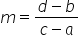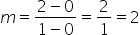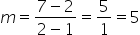Maths-
General
Easy

Question

# Given an example of a non-linear function in table form. X           YHint:

## The correct answer is: not a linear function.

### Step by step solution:We take the values of x to 0, 1, 2, 3 and 4.First, we take the value as y = 0 for x = 0Next, we assume y = 2 for x = 1The slope between two points (a, b) and (c, d) is given byWe take the above two points to be(a, b) = (0, 0) and (c, d) = (1, 2)Calculating the slope, we haveThus, the slope between first two points = 2Next, for x = 2, we choose the value of y in such a way that the slope between the second point and this point is not equal to 2.For x = 2, we choose, y = 7Slope between two points (a, b) and (c, d) is given byWe take the above two points to be(a, b) = (1, 2) and (c, d) = (2, 7)Calculating the slope, we haveFor the rest of the values of x, we can choose any arbitrary value of y.We choose,For x = 3, y = 4For x = 4, y = 9Finally, the table becomes x 0 1 2 3 4 y 0 2 7 4 9 This is an example of a non linear function.

We know that the graph of a non linear function is not a straight line.
So, we can plot the above ordered pairs to verify that the graph of the above points is not a straight line and hence not a linear function.#### With Turito Foundation.#### Get an Expert Advice From Turito.